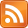Here are several equations.

$$\frac{1}{2} + \frac{1}{6} = \frac{2}{3}$$ $$\frac{2}{3} + \frac{1}{12} = \frac{3}{4}$$ $$\frac{3}{4} + \frac{1}{20} = \frac{4}{5}$$ $$\frac{4}{5} + \frac{1}{30} = \frac{5}{6}$$

Looking at them, one might ask:

• What's the next equation?
• Why?
• (Bonus) What's the equation that comes before the first one on the list?

These all have answers. You should* spend a few minutes thinking about them yourself. Click here to see my work, which attempts in two ways to explain what's going on.

* I mean, you should do whatever will make you happy and doesn't hurt others.